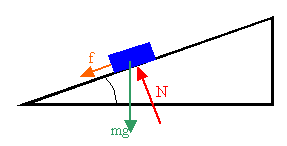d – Job Exam Rare Mathematics (JEMrare) - Solved MCQs | Job Exam Rare Mathematics (JEMrare) - Solved MCQsLet Reaction force be =N

Let angle at which road is banked =Ncosθ = mg (horizontal component of N is balancing weight of the car) –1(1)

and

Nsinθ = mv2/r (Vertical component of N is balancing centrifugal force on the car)–(2)

Dividing eq 2 by eq 1 we get

tanθ = v2/(gr)